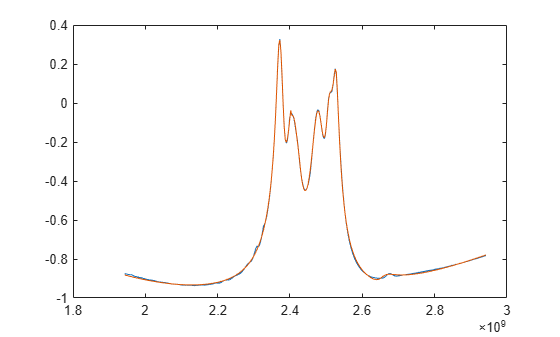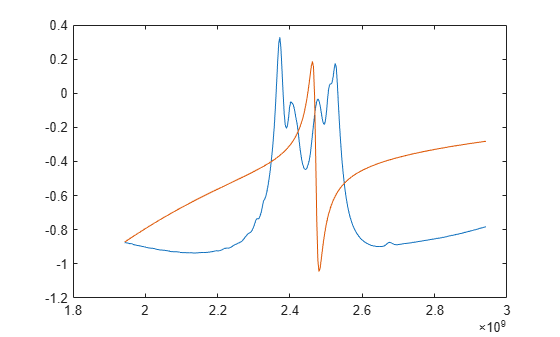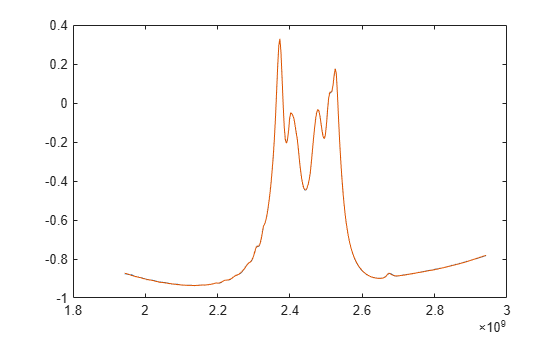Using Rational Object to Fit S-Parameters

This example shows how to use the rational object to create a rational fit to S-parameter data, and the various properties and methods that are included in the rational object.

Create rational object

Read in the sparameters, and create the rational object from them. The rational function automatically fits all entries of the S-parameter matrices.

S = sparameters('sawfilter.s2p')
S =
sparameters: S-parameters object

NumPorts: 2
Frequencies: [334x1 double]
Parameters: [2x2x334 double]
Impedance: 50

rfparam(obj,i,j) returns S-parameter Sij

r = rational(S)
r =
rational with properties:

NumPorts: 2
NumPoles: 36
Poles: [36x1 double]
Residues: [2x2x36 double]
DirectTerm: [2x2 double]
ErrDB: -40.8708

With the default settings on this example, the rational function achieves an accuracy of about -26 dB, using 30 poles. By construction, the rational object is causal, with a non-zero direct term.

Compare fit with original data

Generate the frequency response from the rational object, and compare one of the entries with the original data.

resp = freqresp(r, S.Frequencies);
plot(S.Frequencies, real(rfparam(S, 1, 1)), ...
S.Frequencies, real(squeeze(resp(1,1,:))))Limit number of poles

Redo the fit, limiting the number of poles to a maximum of 5. The rational object may use fewer poles than specified. Notice that the quality of the fit is degraded as opposed to the original 30-pole fit.

r5 = rational(S, 'MaxPoles', 5)
r5 =
rational with properties:

NumPorts: 2
NumPoles: 4
Poles: [4x1 double]
Residues: [2x2x4 double]
DirectTerm: [2x2 double]
ErrDB: -0.7603

resp5 = freqresp(r5, S.Frequencies);
plot(S.Frequencies, real(rfparam(S, 1, 1)), ...
S.Frequencies, real(squeeze(resp5(1,1,:))))Tighten target accuracy

Redo the fit, asking for a tighter tolerance (-60dB), Notice that the fit is significantly improved, particularly in the stopbands of the sawfilter.

rgood = rational(S, -60)
rgood =
rational with properties:

NumPorts: 2
NumPoles: 101
Poles: [101x1 double]
Residues: [2x2x101 double]
DirectTerm: [2x2 double]
ErrDB: -54.4408

respgood = freqresp(rgood, S.Frequencies);
plot(S.Frequencies, real(rfparam(S, 1, 1)), ...
S.Frequencies, real(squeeze(respgood(1,1,:))))